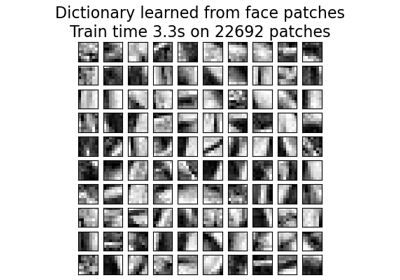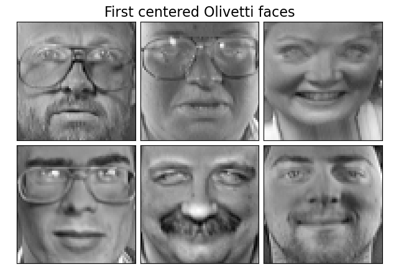sklearn.decomposition.MiniBatchDictionaryLearning¶

class sklearn.decomposition.MiniBatchDictionaryLearning(n_components=None, *, alpha=1, n_iter=1000, fit_algorithm='lars', n_jobs=None, batch_size=3, shuffle=True, dict_init=None, transform_algorithm='omp', transform_n_nonzero_coefs=None, transform_alpha=None, verbose=False, split_sign=False, random_state=None, positive_code=False, positive_dict=False, transform_max_iter=1000)[source]

Mini-batch dictionary learning

Finds a dictionary (a set of atoms) that can best be used to represent data using a sparse code.

Solves the optimization problem:

(U^*,V^*) = argmin 0.5 || Y - U V ||_2^2 + alpha * || U ||_1
(U,V)
with || V_k ||_2 = 1 for all  0 <= k < n_components

Read more in the User Guide.

Parameters
n_componentsint,

number of dictionary elements to extract

alphafloat,

sparsity controlling parameter

n_iterint,

total number of iterations to perform

fit_algorithm{‘lars’, ‘cd’}

lars: uses the least angle regression method to solve the lasso problem (linear_model.lars_path) cd: uses the coordinate descent method to compute the Lasso solution (linear_model.Lasso). Lars will be faster if the estimated components are sparse.

n_jobsint or None, optional (default=None)

Number of parallel jobs to run. None means 1 unless in a joblib.parallel_backend context. -1 means using all processors. See Glossary for more details.

batch_sizeint,

number of samples in each mini-batch

shufflebool,

whether to shuffle the samples before forming batches

dict_initarray of shape (n_components, n_features),

initial value of the dictionary for warm restart scenarios

transform_algorithm{‘lasso_lars’, ‘lasso_cd’, ‘lars’, ‘omp’, ‘threshold’}

Algorithm used to transform the data. lars: uses the least angle regression method (linear_model.lars_path) lasso_lars: uses Lars to compute the Lasso solution lasso_cd: uses the coordinate descent method to compute the Lasso solution (linear_model.Lasso). lasso_lars will be faster if the estimated components are sparse. omp: uses orthogonal matching pursuit to estimate the sparse solution threshold: squashes to zero all coefficients less than alpha from the projection dictionary * X’

transform_n_nonzero_coefsint, 0.1 * n_features by default

Number of nonzero coefficients to target in each column of the solution. This is only used by algorithm='lars' and algorithm='omp' and is overridden by alpha in the omp case.

transform_alphafloat, 1. by default

If algorithm='lasso_lars' or algorithm='lasso_cd', alpha is the penalty applied to the L1 norm. If algorithm='threshold', alpha is the absolute value of the threshold below which coefficients will be squashed to zero. If algorithm='omp', alpha is the tolerance parameter: the value of the reconstruction error targeted. In this case, it overrides n_nonzero_coefs.

verbosebool, optional (default: False)

To control the verbosity of the procedure.

split_signbool, False by default

Whether to split the sparse feature vector into the concatenation of its negative part and its positive part. This can improve the performance of downstream classifiers.

random_stateint, RandomState instance or None, optional (default=None)

Used for initializing the dictionary when dict_init is not specified, randomly shuffling the data when shuffle is set to True, and updating the dictionary. Pass an int for reproducible results across multiple function calls. See Glossary.

positive_codebool

Whether to enforce positivity when finding the code.

New in version 0.20.

positive_dictbool

Whether to enforce positivity when finding the dictionary.

New in version 0.20.

transform_max_iterint, optional (default=1000)

Maximum number of iterations to perform if algorithm='lasso_cd' or lasso_lars.

New in version 0.22.

Attributes
components_array, [n_components, n_features]

components extracted from the data

inner_stats_tuple of (A, B) ndarrays

Internal sufficient statistics that are kept by the algorithm. Keeping them is useful in online settings, to avoid losing the history of the evolution, but they shouldn’t have any use for the end user. A (n_components, n_components) is the dictionary covariance matrix. B (n_features, n_components) is the data approximation matrix

n_iter_int

Number of iterations run.

iter_offset_int

The number of iteration on data batches that has been performed before.

random_state_RandomState

RandomState instance that is generated either from a seed, the random number generattor or by np.random.

Notes

References:

J. Mairal, F. Bach, J. Ponce, G. Sapiro, 2009: Online dictionary learning for sparse coding (https://www.di.ens.fr/sierra/pdfs/icml09.pdf)

Methods

 fit(X[, y]) Fit the model from data in X. fit_transform(X[, y]) Fit to data, then transform it. get_params([deep]) Get parameters for this estimator. partial_fit(X[, y, iter_offset]) Updates the model using the data in X as a mini-batch. set_params(**params) Set the parameters of this estimator. transform(X) Encode the data as a sparse combination of the dictionary atoms.
__init__(n_components=None, *, alpha=1, n_iter=1000, fit_algorithm='lars', n_jobs=None, batch_size=3, shuffle=True, dict_init=None, transform_algorithm='omp', transform_n_nonzero_coefs=None, transform_alpha=None, verbose=False, split_sign=False, random_state=None, positive_code=False, positive_dict=False, transform_max_iter=1000)[source]

Initialize self. See help(type(self)) for accurate signature.

fit(X, y=None)[source]

Fit the model from data in X.

Parameters
Xarray-like, shape (n_samples, n_features)

Training vector, where n_samples in the number of samples and n_features is the number of features.

yIgnored
Returns
selfobject

Returns the instance itself.

fit_transform(X, y=None, **fit_params)[source]

Fit to data, then transform it.

Fits transformer to X and y with optional parameters fit_params and returns a transformed version of X.

Parameters
X{array-like, sparse matrix, dataframe} of shape (n_samples, n_features)
yndarray of shape (n_samples,), default=None

Target values.

**fit_paramsdict

Returns
X_newndarray array of shape (n_samples, n_features_new)

Transformed array.

get_params(deep=True)[source]

Get parameters for this estimator.

Parameters
deepbool, default=True

If True, will return the parameters for this estimator and contained subobjects that are estimators.

Returns
paramsmapping of string to any

Parameter names mapped to their values.

partial_fit(X, y=None, iter_offset=None)[source]

Updates the model using the data in X as a mini-batch.

Parameters
Xarray-like, shape (n_samples, n_features)

Training vector, where n_samples in the number of samples and n_features is the number of features.

yIgnored
iter_offsetinteger, optional

The number of iteration on data batches that has been performed before this call to partial_fit. This is optional: if no number is passed, the memory of the object is used.

Returns
selfobject

Returns the instance itself.

set_params(**params)[source]

Set the parameters of this estimator.

The method works on simple estimators as well as on nested objects (such as pipelines). The latter have parameters of the form <component>__<parameter> so that it’s possible to update each component of a nested object.

Parameters
**paramsdict

Estimator parameters.

Returns
selfobject

Estimator instance.

transform(X)[source]

Encode the data as a sparse combination of the dictionary atoms.

Coding method is determined by the object parameter transform_algorithm.

Parameters
Xarray of shape (n_samples, n_features)

Test data to be transformed, must have the same number of features as the data used to train the model.

Returns
X_newarray, shape (n_samples, n_components)

Transformed data

Examples using sklearn.decomposition.MiniBatchDictionaryLearning¶